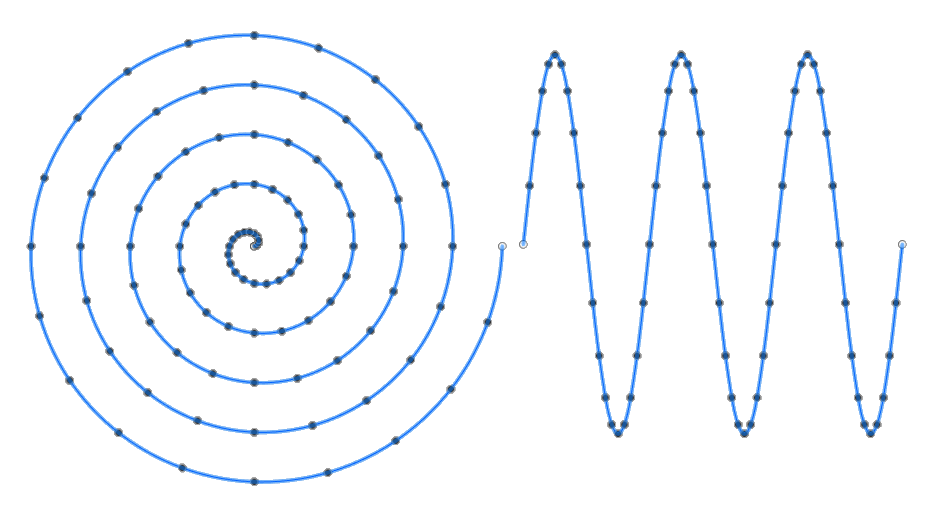# Creating Equation Curves in Fusion 360Unlike Inventor, Fusion 360 doesn’t support the ability to create a spline based on an equation.  However, using the Fusion 360 API you can create close approximations of equation curves.  You do this by creating a spline through a series of points.  You write code that uses the equation to define the X, Y, and Z coordinates of the points.

Because the points represent a sampling of coordinates along the curve, the result is an approximation, but in most cases is still very close.  You can increase the number of sample points to get a more accurate result but this will result in a more complex curve.  More complex curves will slow down any downstream processes that use the curve.  There’s a balancing point where you want to use as few points as possible but still get the desired accuracy.  The position of the points along the curve is also important.  For example, when computing a spline curve, you want to have a sample point at the peak of the amplitude so you capture the full height of the curve.

### Example 1 (Archimedean Spiral)

Here are a couple of examples that illustrate how this works.  The first one is based on a question in the Fusion 360 API Forum, which prompted me to create this post.  The equation is one for an Archimedean spiral.  From the Wikipedia article you’ll see that the equation is:

r = a + bθ

And here’s my conversion of that equation into some code that creates a series of points along the equation curve and then creates a spline using those points.  In lines 16-20, I’m defining the various inputs for the equation and some other settings to control the number of points that are generated.  In lines 21-25, I’m looping to create the points based on the equation.  And finally, in line 29 it creates the fitted spline using the points.

```
def archimedeanSpiral():
ui = None
try:
ui  = app.userInterface

root = des.rootComponent

# Create a new sketch.

# Create a series of points along the spiral using the spiral equation.
# r = a + (beta * theta)
numTurns = 5
pointsPerTurn = 20
distanceBetweenTurns = 5  # beta
theta = 0
offset = 5                # a
for i in range(pointsPerTurn * numTurns + 1):
r = offset + (distanceBetweenTurns * theta)
x = r * math.cos(theta)
y = r * math.sin(theta)

theta += (math.pi*2) / pointsPerTurn

except:
if ui:
ui.messageBox('Failed:\n{}'.format(traceback.format_exc()))
```

### Example 2 (Sin Wave)

Here’s a very similar program but it uses the sin function as the equation to drive the curve.  Hopefully, you can see that it should be possible to incorporate any equation.

```
def sinCurve():
ui = None
try:
ui  = app.userInterface

root = des.rootComponent

# Create a new sketch.

# Create a series of points along the sin wave.
amplitude = 120
frequency = 80
numWaves = 3
pointsPerWave = 20
alpha = 0
x = 0
for i in range(pointsPerWave*numWaves + 1):
y = amplitude * math.sin(alpha)

x += frequency / pointsPerWave
alpha += (math.pi*2) / pointsPerWave

except:
if ui:
ui.messageBox('Failed:\n{}'.format(traceback.format_exc()))
```

### 3D Curves

The two previous examples created 2D curves but that’s only because I set the Z component of the point to be zero. I could just have easily set a Z value to create a 3D curve too.  Below is a section of the Archimedean Spiral example where the Z value is decreasing for each point, so the center portion of the spiral protrudes.

```
z = 0
for i in range(pointsPerTurn * numTurns + 1):
r = offset + (distanceBetweenTurns * theta)
x = r * math.cos(theta)
y = r * math.sin(theta)

theta += (math.pi*2) / pointsPerTurn
z += -3
```

Below is a model created from the resulting spline by lofting between two circles and using the spline as a guide.### 7 thoughts on “Creating Equation Curves in Fusion 360”

1.Thank you for this post!
I am surprised Fusion does not have a native tool to do this because the script is pretty simple. It is what I needed though so thank you.

2.I could not access the Fusion 360 API. How do I access it?

•The API comes with Fusion and all of the documentation is online. If you click the help button in the upper-right corner of Fusion there’s a “Learning and Documentation” link and under that is “Fusion 360 API”. Here’s a direct link.

3.Thank you, Brian. I found the API under the Tools tab.

Now I’m trying to create my own equations and debug the scripts. I tried running the examples above, but I got no output and no error messages. What do I do next?

•Not sure if this is the answer you’re looking for, but these scripts merely define the functions. Create a new python script in Fusion360. It’s merely a hello world dialog box, but it’s good boilerplate code that works.

For the archimedian spiral

Make sure the include line has math in it:

there should be a ui.messagebox(‘Hello script’) line, add this right after it:
archimedeanSpiral()

A few lines below that, copy and paste the whole def archimedeanSpiral(): block of code.

Save the file and run it.

4.